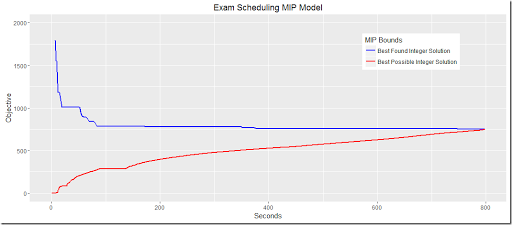## Wednesday, March 23, 2016

### ggplot and miptrace

The output of miptrace can be used to plot the MIP bounds. In this post we used it to show the results on an Exam Scheduling Model. After a little bit of clean-up here is a better version:

```plot.trace <- function(filename,ylimits,title=basename(filename)) {
hdr<-c("lineNum", "seriesID", "node", "Seconds", "bnd1", "bnd2")
library(tidyr)
df<-gather(df,key=bound,value=Objective,bnd1,bnd2)
p<-ggplot(data=df,aes(x=Seconds))+geom_line(aes(y=Objective,color=bound),size=1.03)+
ggtitle(title)+theme(legend.position=c(.8, .8))+
scale_color_manual(name="MIP Bounds",values=c("blue","red"),
labels=c("Best Found Integer Solution","Best Possible Integer Solution"))
if (!missing(ylimits)) {
p<-p+ylim(ylimits)
}
return(p)
}
plot.trace("c:/tmp/exam3.csv",c(0,2000),"Exam Scheduling MIP Model")```

We pivot the data into long format (“collapsing” the last two columns with the bounds into a key, value pair of columns). The output looks like: